# 單元測試：Mocha、Chai 和 Sinon

## 單元測試（Unit Test）

### 什麼是 Unit Test？

``````// 給定輸入為 1 和 2

// 預期得到輸出 3
if (result !== 3) {
throw new Error('Failed');
}
``````

### 為什麼要做 Unit Test？

• 防止改 A 壞 B，避免不能跑的程式碼比能跑的還多
• 明確指出問題所在、告知正確的行為是什麼，減少猜測時間

### 如何做 Unit Test？

• 使用 npm 等安裝 Mocha、Chai 和 Sinon 並 require。
• 撰寫測試程式。
• 使用 mocha 啟動測試。
• 檢視測試報告，確認通過或未通過的項目。
• 根據測試結果進行調整：對通過的程式碼做重構，或對未通過的程式碼除錯。

## Mocha

Mocha 是一個 JavaScript 的測試框架，目的是用來管理測試的程式碼。

### 語法說明

• `describe()`：描述場景或圈出特定區塊，例如：標明測試的功能或 function。
• `it()`：撰寫測試案例（Test Case）。
• `before()`：在所有測試開始前會執行的程式碼區塊。
• `after()`：在所有測試結束後會執行的程式碼區塊。
• `beforeEach()`：在每個 Test Case 開始前執行的程式碼區塊。
• `afterEach()`：在每個 Test Case 結束後執行的程式碼區塊。

### 語法範例

``````describe('hooks', function() {
// 測試區塊
before(function() {
// 在所有測試開始前會執行的程式碼區塊
});

after(function() {
// 在所有測試結束後會執行的程式碼區塊
});

beforeEach(function() {
// 在每個 Test Case 開始前執行的程式碼區塊
});

afterEach(function() {
// 在每個 Test Case 結束後執行的程式碼區塊
});

// 撰寫個別 Test Case
it('should ...', function() {
// 執行 Test Case
});
});
``````

### TDD vs BDD

TDD BDD

Test-Driven Development

Behavior Driven Development

## Chai

Chai 提供 BDD 語法測試用的斷言庫（Assertion Library）。斷言庫是一種判斷工具，驗證執行結果是否符合預期，若實際結果和預測不同，就是測到 bug 了。以下分 Assert 和 Expect / Should 說明。

### Assert

`assert(expression, message)`：測試這個項目的 expression `'foo' === 'bar'` 是否為真，若為假則顯示錯誤訊息 message。

``````var assert = chai.assert;

describe('AssertTest', function() {
var foo = 'Hello';
var bar = 'World';

it('should be equal', function() {
assert('foo' === 'bar', 'foo is not bar');
});
});
``````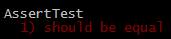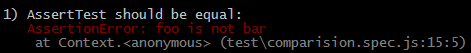### Expect / Should

``````var expect = chai.expect;

describe('ExpectTest', function() {
it('should be equal', function() {
expect(3).to.equal(2);
});
});
``````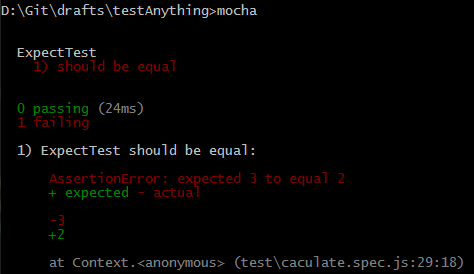``````// calculateModule
return x + y;
}

function _sub(x, y) {
return x - y;
}

module.exports = {
sub: _sub,
};
``````
``````const expect = require('chai').expect;
const testModule = require('../modules/calculateModule');

it('1 + 2 = 3', () => {
});

it('3 + 4 = 7', () => {
});
});

describe('測試 sub', () => {
it('1 - 2 = -1', () => {
expect(testModule.sub(1, 2)).to.equal(-1);
});

it('11 - 4 = 7', () => {
expect(testModule.sub(11, 4)).to.equal(7);
});
});
``````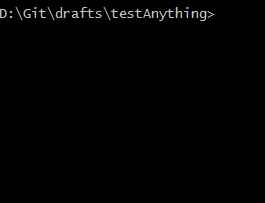### 比較 Assert、Expect、Should 的差異

• Should 會修改 Object.prototype
• Should 在瀏覽器環境下，對 IE 有相容問題
• Should 無法客製化錯誤訊息
``````// Assert 和 Expect 的客製化錯誤訊息範例
assert.isTrue(foo, 'foo should be true');
expect(foo, 'foo should be true').to.be.true;
``````

## Sinon

### Spy

``````const expect = require('chai').expect;
const sinon = require('sinon');
const testModule = require('../modules/comparisionModule');

describe('測試 comparePelple', function() {
it('should call the callback function', function() {
var nameList = ['Nina', 'Ricky'];
var callback = sinon.spy();

testModule.comparePelple(nameList, nameList, callback);
console.log(callback.callCount); // 該 function 被呼叫的次數
});
});
``````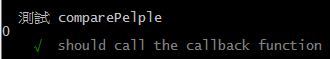### Stub

#### 範例

``````const expect = require('chai').expect;
const sinon = require('sinon');
const uuid = require('node-uuid');

describe('stub example', () => {
it('check length of uuid ', () => {
var stub = sinon.stub(uuid, 'v4');
var mockId = stub.v4();
expect(mockId.length).to.equal(36);
uuid.v4.restore();
});
});
``````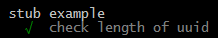#### 範例：Ajax Request

``````function saveUser(user, callback) {
\$.ajax(
'/users',
{
first: user.firstname,
last: user.lastname,
},
callback,
);
}

describe('Stub: ajax', () => {
it('should call callback after saving', () => {
var ajax = sinon.stub(\$, 'ajax'); // 取代 ajax function，並不會真的呼叫
ajax.yields('Hello', 'World'); // 準備把傳入 callback 的參數 ['Hello', 'World'] 丟進去

var callback = sinon.spy();
saveUser({ firstname: 'Han', lastname: 'Solo' }, callback);

ajax.restore(); // 清除 test double
expect(callback.callCount).to.equal(777); // 該 function 被呼叫的次數
});
});
``````

### Mock

``````var opts = {
call: function(msg) {
console.log(msg);
},
};

describe('Mock', () => {
it('should pass Hello World to run call()', function() {
var mock = sinon.mock(opts);
mock
.expects('call')
.once()
.withExactArgs('Hello World');
opts.call('Hello World');
mock.restore();
mock.verify();
});
});
``````

### 使用時機

• Spy：用於監看或驗證。
• Stub：使用一些假資料，測試各種狀況下功能的運作狀況。
• Mock：除了上述 Spy 和 Stub 的情況外，還需隔絕測試過程於完全獨立的環境。

### Chai Assertion vs Sinon Assertion

Chai Assertion 報錯範例。

``````assert('foo' === 'bar');
``````

``````AssertionError: Unspecified AssertionError
``````

``````assert('foo' === 'bar', 'foo is not bar');
``````
``````AssertionError: foo is not bar
``````

Sinon Assertion 報錯範例。

``````var expected = { x: 999 },
actual = { x: 1, y: 2 };
sinon.assert.match(actual, expected);
``````

``````AssertError: expected value to match
expected = { x: 999 }
actual = { x: 1, y: 2 }
``````